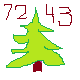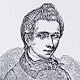Discussion about math, puzzles, games and fun.   Useful symbols: ÷ × ½ √ ∞ ≠ ≤ ≥ ≈ ⇒ ± ∈ Δ θ ∴ ∑ ∫  π  -¹ ² ³ °

You are not logged in.

## #1 2019-05-18 03:50:26

John E. Franklin(2)
MemberRegistered: 2019-05-17
Posts: 20

### Adding 3 Numbers -- Easy Stuff

1.) 1 + 2 + 3 =

2.) 4 + 5 + 13 =

3.) 0 + 1 + 2 =

4.) 5 + 7 + 10 =

5.) 6 + 7 + 9 =

6.) 4 + 5 + 7 =

7.) 1 + 3 + 5 =

8.) 2 + 4 + 6 =

9.) 4 + 5 + 7 =

10.) 2 + 2 + 3 =

11.) 13 + 14 + 15 =

12.) 12 + 13 + 15 =

13.) 9 + 13 + 19 =

14.) 3 + 3 + 3 =

15.) 1 + 4 + 7 =

16.) 1 + 3 + 6 =

17.) 11 + 18 + 25 =

18.) 3 + 6 + 9 =

19.) 2 + 6 + 10 =

20.) 1 + 1 + 4 =

21.) 1 + 6 + 7 =

22.) 4 + 6 + 6 =

23.) 5 + 7 + 8 =

24.) 7 + 9 + 9 =

25.) 2 + 2 + 4 =

26.) 3 + 8 + 9 =

27.) 2 + 3 + 4 =

28.) 3 + 4 + 9 =

29.) 9 + 12 + 14 =

30.) 4 + 6 + 7 =

31.) 5 + 8 + 11 =

32.) 4 + 8 + 8 =

33.) 6 + 6 + 12 =

34.) 4 + 17 + 56 =

35.) 22 + 33 + 44 =

36.) 40 + 41 + 44 =

37.) 2 + 3 + 6 =

38.) 3 + 3 + 4 =

39.) 1 + 1 + 1 =

40.) 1 + 1 + 5 =

41.) 3 + 5 + 6 =

42.) 4 + 9 + 19 =

43.) 1 + 2 + 4 =

44.) 4 + 6 + 8 =

45.) 5 + 8 + 9 =

46.) 6 + 8 + 9 =

47.) 8 + 12 + 25 =

48.) 3 + 5 + 9 =

49.) 11 + 26 + 88 =

50.) 0 + 0 + 2 =

Last edited by John E. Franklin(2) (2019-05-18 14:32:30)

Offline

## #2 2019-05-18 14:41:29

ganeshRegistered: 2005-06-28
Posts: 31,672

### Re: Adding 3 Numbers -- Easy Stuff

Hi John E. Franklin,

Relatively simple.

It is no good to try to stop knowledge from going forward. Ignorance is never better than knowledge - Enrico Fermi.

Nothing is better than reading and gaining more and more knowledge - Stephen William Hawking.

Offline

## #3 2019-05-18 15:03:52

John E. Franklin(2)
MemberRegistered: 2019-05-17
Posts: 20

### Re: Adding 3 Numbers -- Easy Stuff

Check out #49 for why you say 85?

Offline

## #4 2019-05-18 15:22:19

ganeshRegistered: 2005-06-28
Posts: 31,672

### Re: Adding 3 Numbers -- Easy Stuff

Corrected :

An error crept in. Conceded.

It is no good to try to stop knowledge from going forward. Ignorance is never better than knowledge - Enrico Fermi.

Nothing is better than reading and gaining more and more knowledge - Stephen William Hawking.

Offline

## #5 2019-05-19 04:10:18

John E. Franklin(2)
MemberRegistered: 2019-05-17
Posts: 20

### Re: Adding 3 Numbers -- Easy Stuff

Good Job, ganesh.  From John.

Offline

## #6 2019-05-19 04:29:17

John E. Franklin(2)
MemberRegistered: 2019-05-17
Posts: 20

### Re: Adding 3 Numbers -- Easy Stuff

51.) 1 + 3 + 5 =

52.) 2 + 4 + 6 =

53.) 3 + 5 + 7 =

54.) 4 + 6 + 8 =

55.) 5 + 7 + 9 =

56.) 45 + 65 + 85 =

57.) 32 + 40 + 66 =

58.) 2 + 12 + 22 =

59.) 1 + 14 + 28 =

60.) 3 + 115 + 117 =

Offline

## #7 2019-05-19 05:07:04

John E. Franklin(2)
MemberRegistered: 2019-05-17
Posts: 20

### Re: Adding 3 Numbers -- Easy Stuff

Press button to get the answer.

Last edited by John E. Franklin(2) (2019-05-19 06:41:42)

Offline

## #8 2019-05-19 07:07:44

John E. Franklin(2)
MemberRegistered: 2019-05-17
Posts: 20

Offline

## #9 2019-05-19 08:00:00

John E. Franklin(2)
MemberRegistered: 2019-05-17
Posts: 20

### Re: Adding 3 Numbers -- Easy Stuff

Push a button to see the answer.

Offline

## #10 2019-05-19 08:56:46

John E. Franklin(2)
MemberRegistered: 2019-05-17
Posts: 20

Offline

## #11 2019-05-19 09:15:06

Alg Num Theory
MemberRegistered: 2017-11-24
Posts: 693
Website

### Re: Adding 3 Numbers -- Easy Stuff

Do you have more interesting exercises than just adding three numbers?

Me, or the ugly man, whatever (3,3,6)

Offline

## #12 2019-05-19 09:25:25

John E. Franklin(2)
MemberRegistered: 2019-05-17
Posts: 20

### Re: Adding 3 Numbers -- Easy Stuff

I am quite happy with each and every three number set.
Thanks for being concerned though.

Offline

## #13 2019-05-19 10:37:55

John E. Franklin(2)
MemberRegistered: 2019-05-17
Posts: 20

Offline

## #14 2019-05-19 12:38:50

ganeshRegistered: 2005-06-28
Posts: 31,672

### Re: Adding 3 Numbers -- Easy Stuff

Hi John E. Franklin(2),

It is no good to try to stop knowledge from going forward. Ignorance is never better than knowledge - Enrico Fermi.

Nothing is better than reading and gaining more and more knowledge - Stephen William Hawking.

Offline

## #15 2019-05-19 22:52:07

John E. Franklin(2)
MemberRegistered: 2019-05-17
Posts: 20

### Re: Adding 3 Numbers -- Easy Stuff

Almost perfect.  Just a tiny error in 66 + 660 + 6600...

Offline